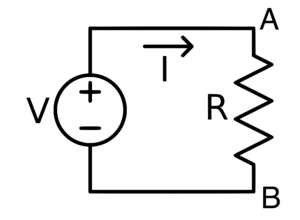# Electric Potential and Potential Difference Notes - Class 10

## Class 10: Electric Potential and Potential Difference Notes - Class 10

The document Electric Potential and Potential Difference Notes - Class 10 is a part of Class 10 category.
All you need of Class 10 at this link: Class 10

## Electric Potential

If two charged bodies are in contact, the charge starts flowing from one conductor to other. The electric condition, that determines the flow of charge from one conductor to other in contact, is the electric potential. Electric potential is comparable to level in case of water, the temperature in case of heat and pressure in the case of fluids. Earth is a conductor that can hold an infinite charge and can give infinite charge without changing its potential.

Its potential is taken as zero potential. Thus the positively charged conductor has more positive potential than the earth. A  negatively charged conductor has negative potential less than that of the earth.The electric potential difference between two points in an electric circuit is the work done in moving a unit positive charge from one point to the other.

When W joule of work is done in bringing the test charge Q coulomb from infinity to the point P then the electric potential V at the point P then, V=  W/Q

The work needed to move a charge Q from infinity to a point P where electric potential is  V will be W=QV

The S.I unit of work is joule and that of the charge is coulomb.

## Potential differenceWhen the current flows between two points A and B of an electric circuit, we only consider the charge between the points A and B, this means it is not necessary to know the exact potential at each point A and B. It is sufficient to know the potential difference between the two points A and B. Therefore the potential difference  between the two points is equal to the work done in moving a unit positive charge from one point to the other.

The potential difference is expressed in volt (V).  The potential difference between two points is said to be 1 volt if the work is done in moving 1-coulomb charge from one point to other is 1 joule.

1 volt= 1 joule/ 1 coulomb

Unit for measuring the potential difference is volt and instrument used for measuring potential difference is a voltmeter. While connecting voltmeter in the circuit, positive terminal of the voltmeter should be in connection with the positive terminal of the cell and negative with the negative of the cell.

### What happens when the battery is in connection with the circuit?

When a conducting wire is in connection with the terminals of the battery, a potential difference is created between the ends of the conductor. This potential difference sets up an electric field throughout the conductor.The electrons near the positive terminal of the battery are attracted by it and start on the move towards positive terminal.

The document Electric Potential and Potential Difference Notes - Class 10 is a part of Class 10 category.
All you need of Class 10 at this link: Class 10Use Code STAYHOME200 and get INR 200 additional OFF

Track your progress, build streaks, highlight & save important lessons and more!

,

,

,

,

,

,

,

,

,

,

,

,

,

,

,

,

,

,

,

,

,

;